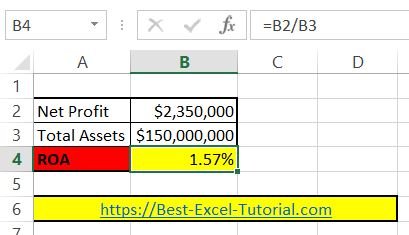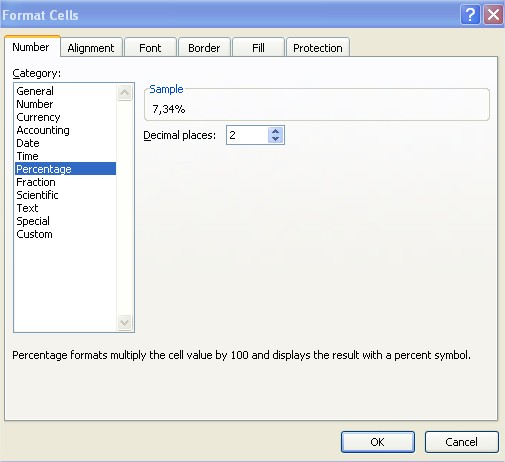#### How to calculate ROA?

In this Excel tutorial lesson, you will learn how to calculate ROA in Excel.

## What is ROA?

ROA stands for return on assets. Return of assets is one of the most basic measures in business. ROA is an indicator which is used to measure the efficiency of a company.

Let’s build the return on assets calculator in Excel. To calculate ROA in Excel, first you need some data. You need net profit and total assets.

Copy and paste this roa formula in cell B4: =B2/B3## ROA calculator

This formula will calculate the ROA for the assets data you place in cells B2 and B3 and is based on the roa equation formula:

ROA = Net Profit / Total Assets

Remember to format ROA as Percentage. Click B4 cell > click CTRL + 1 keyboard shortcut > click Percentage with 2 decimal places.Note: The net income and total assets figures should be for the same time period, such as a quarter or a year, to ensure an accurate comparison.